# Frank solutions for Class 9 Physics ICSE chapter 8 - Electricity and Magnetism [Latest edition]

#### Chapters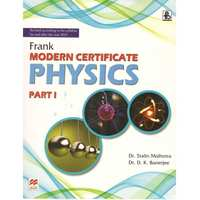## Chapter 8: Electricity and Magnetism

Static ElectricityCurrent ElectricityExercise
Static Electricity [Pages 300 - 302]

### Frank solutions for Class 9 Physics ICSE Chapter 8 Electricity and Magnetism Static Electricity [Pages 300 - 302]

Static Electricity | Q 1 | Page 300
What do you mean by electrostatics?
Static Electricity | Q 2 | Page 300
What is the other name of frictional electricity?
Static Electricity | Q 3 | Page 300
Name the scientist who first observed the electrical properties of amber.
Static Electricity | Q 4 | Page 300
How many kinds of charges have you come across?
Static Electricity | Q 5 | Page 300
What does a positively charged body signify?
Static Electricity | Q 6 | Page 300
What does a negatively charged body signify?
Static Electricity | Q 7 | Page 300
Name the scientist who first assigned algebraic signs to charges.
Static Electricity | Q 8 | Page 300
What will happen when a glass rod is rubbed with some silk cloth?
Static Electricity | Q 9 | Page 300
What type of charge is produced on an ebonite rod when it is rubbed with a cat's fur?
Static Electricity | Q 10 | Page 300
What is the quantization of charge?
Static Electricity | Q 11 | Page 300
Name a physical quantity, other than an electric charge, which is quantized.
Static Electricity | Q 12 | Page 300
How many electrons are present in one coulomb?
Static Electricity | Q 13 | Page 301
Which is bigger: 1 C or magnitude of the charge on an electron?
Static Electricity | Q 14 | Page 301
What is the value of charge on a body which carries 100 excess electrons?
Static Electricity | Q 15 | Page 301
Is a charge of -3.6 X 10-18C possible?
Static Electricity | Q 16 | Page 301
What is the origin of frictional forces?
Static Electricity | Q 17 | Page 301
What is the cause of charging?
Static Electricity | Q 18 | Page 301
Who first showed two kinds of charges and who gave them algebraic signs?
Static Electricity | Q 19 | Page 301
State two differences between charge and mass.
Static Electricity | Q 20 | Page 301
Is the mass of the body affected by charging?
Static Electricity | Q 21 | Page 301
Consider three charged bodies A, B, and C. If A and B repel each other and A attracts C, what is the nature of force between B and C?
Static Electricity | Q 22 | Page 301
How do the charges interact?

Static Electricity | Q 23 | Page 301
Does the motion of a body affect its charge?
Static Electricity | Q 24 | Page 301
Name any two basic properties of electric charge.
Static Electricity | Q 25 | Page 301
Can ever photons have a charge? If not, why?
Static Electricity | Q 26 | Page 301
A glass rod rubbed with silk cloth acquires a charge of +1.6 X 10-12C. What is the charge on the silk cloth?
Static Electricity | Q 27 | Page 301
What is the SI unit of charge?
Static Electricity | Q 28 | Page 301
What is the value of charge on an electron? Is a charge less than the value possible?
Static Electricity | Q 29 | Page 301
What is the net charge on an atom?
Static Electricity | Q 30 | Page 301
What are free electrons?
Static Electricity | Q 32.1 | Page 301

State the kind of charge on a positive ion.

Static Electricity | Q 32.2 | Page 301

State the kind of charge on a negative ion.

Static Electricity | Q 33 | Page 301
Describe an experiment to show that the like charges repel each other while the unlike charges attract.
Static Electricity | Q 34 | Page 301
Sometimes when you take off your nylon clothes, your hair stands on their end. Explain the observation.
Static Electricity | Q 35 | Page 301
The charge on a body is -1.8e. Is this statement true?
Static Electricity | Q 36 | Page 301
Draw a labeled diagram of a gold-leaf electroscope.
Static Electricity | Q 37 | Page 301
What is the purpose of an electroscope?
Static Electricity | Q 38 | Page 302

The following Figure represents a negatively charged gold Ieaf electroscope. Label the parts, P, Q, and R and the estate whether each is an insulator or a conductor. Also, indicate the distribution of charges on the system in the diagram.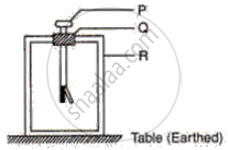Static Electricity | Q 39 | Page 302

The following figure shows a metal rod PQ mounted on an insulated stand. The cap of an uncharged electroscope touches one end Q of the metal rod. A negatively charged ebonite rod is brought near the other end P of the metal rod.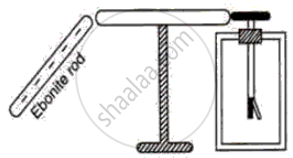(a) What charge does the end P have?
(b) What charge does the end Q have?
(c) What charge does the cap of the electroscope have?
(d) What charge does the gold leaf have?
(e) Will the leaf diverge or collapse? Give reason.
(f) If the electroscope is now earthed, what charge does the metal rod have?

Static Electricity | Q 40 | Page 302
What is meant by the earthing of an electroscope?
Current Electricity [Pages 312 - 313]

### Frank solutions for Class 9 Physics ICSE Chapter 8 Electricity and Magnetism Current Electricity [Pages 312 - 313]

Current Electricity | Q 1 | Page 312

What do you mean by an electric current?

Current Electricity | Q 2 | Page 312

What is an electric cell?

Current Electricity | Q 3 | Page 312

How much is the charge on an electron?

Current Electricity | Q 4 | Page 312

Name the constituents of a cell.

Current Electricity | Q 5.1 | Page 312

Write SI unit of
Electric current,

Current Electricity | Q 5.2 | Page 312

Write SI unit of
Potential difference

Current Electricity | Q 5.3 | Page 312

Write SI unit of
Resistance.

Current Electricity | Q 6 | Page 312

What is the number of electrons that would flow per second through the cross-section of a wire when 1 A current flows in it?

Current Electricity | Q 7 | Page 312

0.7 C charges passes through a cross-section of a conductor in 7 s. Find the current.

Current Electricity | Q 8 | Page 312

Name the instrument used to control current in an electric circuit.

Current Electricity | Q 9 | Page 313

What is the other name of a variable resistor?

Current Electricity | Q 10 | Page 313

What is the function of a switch in an electric circuit?

Current Electricity | Q 11.1 | Page 313

Write symbol and state function of following component in an electric circuit :

cell

Current Electricity | Q 11.2 | Page 313

Write symbol and state function of following component in an electric circuit :

ammeter

Current Electricity | Q 11.3 | Page 313

Write symbol and state function of following component in an electric circuit :

voltmeter

Current Electricity | Q 11.4 | Page 313

Write symbol and state function of following component in an electric circuit : key

Current Electricity | Q 11.5 | Page 313
Write symbol and state function of the following component in an electric circuit :

battery

Current Electricity | Q 11.6 | Page 313

Write symbol and state function of following component in an electric circuit :

rheostat

Current Electricity | Q 12 | Page 313

A current of 1.2 A flows through a conductor for 3.0 s. What amount of charge passes through the conductor?

Current Electricity | Q 13 | Page 313

Label the different parts A, B, C, D, E, and F. State the functions of each part. Show in the diagram the direction of flow of current.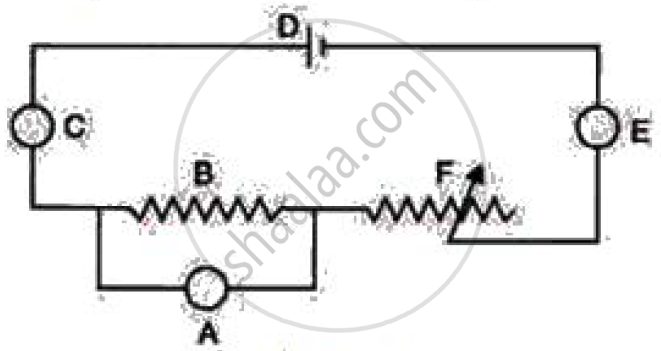Current Electricity | Q 14 | Page 313

l-V graph is shown for a good conductor. What does the slope of the graph represent?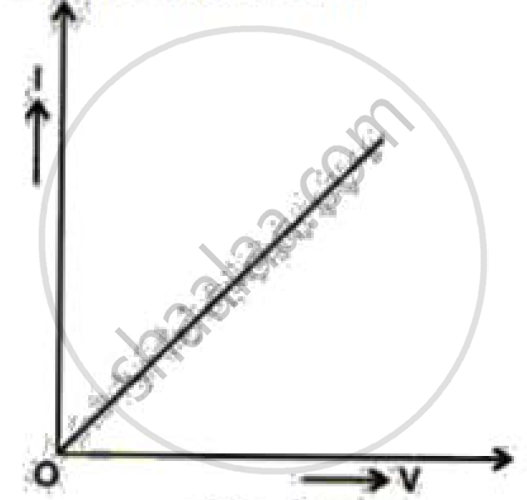Current Electricity | Q 15 | Page 313

What do you mean by the potential difference? Write its SI unit.

Current Electricity | Q 16 | Page 313

Is electric current a scalar quantity?

Current Electricity | Q 17 | Page 313

State the factors on which the electrical resistance of a wire depends.

Current Electricity | Q 18 | Page 313

What is the SI unit of resistance?

Current Electricity | Q 19 | Page 313

A bulb is connected to a cell. How is the resistance of circuit affected if another identical bulb is connected (i) in series, (ii) in parallel, with the first bulb?

Current Electricity | Q 20 | Page 313

How are the potential difference (V), current (l) and resistance (R) related?

Current Electricity | Q 21 | Page 313

'The resistance of a wire is 2 ohm'. Explain the meaning of this statement.

Current Electricity | Q 22.1 | Page 313

Write symbol and state function of following component in an electric circuit :

cell

Current Electricity | Q 22.2 | Page 313

Write symbol and state function of following component in an electric circuit :

ammeter

Current Electricity | Q 22.3 | Page 313

Write symbol and state function of following component in an electric circuit :

voltmeter

Current Electricity | Q 22.4 | Page 313

Write symbol and state function of following component in an electric circuit : key

Current Electricity | Q 22.5 | Page 313
Write symbol and state function of the following component in an electric circuit :

battery

Current Electricity | Q 22.6 | Page 313

Write symbol and state function of following component in an electric circuit :

rheostat

Current Electricity | Q 23 | Page 313

State three factors on which the resistance of a wire depends. Explain, how does the resistance depend on the factors stated by you.

Current Electricity | Q 24 | Page 313

What amount of work is required in moving 3 C charge through a potential difference of 6 V?

Current Electricity | Q 25 | Page 313

What is the physical meaning of resistance?

Current Electricity | Q 26 | Page 313

'The potential difference between two conductors is 1 volt'. Explain the meaning of this statement.

Exercise [Pages 323 - 325]

### Frank solutions for Class 9 Physics ICSE Chapter 8 Electricity and Magnetism Exercise [Pages 323 - 325]

Exercise | Q 1 | Page 323
What are the two kinds of charges?
Exercise | Q 2 | Page 323
What is the net charge on an atom?
Exercise | Q 3 | Page 323
How many electrons are present in Na+? The atomic number of Na is 11.
Exercise | Q 4 | Page 323
Distinguish between an atom and an ion.
Exercise | Q 5 | Page 323
What causes the electrification of two bodies when they are rubbed together?
Exercise | Q 6 | Page 323
What is the function of a lightning conductor?
Exercise | Q 7 | Page 323
The lighting and the thunder are produced simultaneously, but the sound of the thunder is heard a few seconds after the lightning is seen. Why?
Exercise | Q 8 | Page 323
What is the purpose of an electroscope?
Exercise | Q 9 | Page 323
A positively charged rod is brought near the cap of a positively charged gold leaf electroscope. State your observation and give a reason.
Exercise | Q 10 | Page 323
What is direct current?
Exercise | Q 11 | Page 323
Write two differences between the primary and secondary cells.
Exercise | Q 12 | Page 323
What is the SI unit of current?
Exercise | Q 13 | Page 323
What is the difference between a cell and a battery?
Exercise | Q 14 | Page 323
Is current a scalar or a vector quantity?
Exercise | Q 15 | Page 323
Name the physical quantity which defines the rate of flow of charge.
Exercise | Q 16 | Page 323
Which is bigger: a milliampere or a microampere?
Exercise | Q 17 | Page 323
What is the other name for a variable resistor?
Exercise | Q 18 | Page 323
Name two sources of current.
Exercise | Q 19 | Page 323
Is voltmeter same as voltameter?
Exercise | Q 20 | Page 323
Name the instrument used to measure the magnitude of the current flowing in the circuit.
Exercise | Q 21 | Page 323
What is responsible for the flow of current through a metallic conductor?
Exercise | Q 22 | Page 323
Which has a greater resistance: an ammeter or a voltmeter?
Exercise | Q 23 | Page 323
What is an artificial magnet?
Exercise | Q 24 | Page 323
How is a voltmeter connected in a circuit?
Exercise | Q 25 | Page 323
How is an ammeter connected in a circuit?
Exercise | Q 26 | Page 323
Write the SI unit of resistance.
Exercise | Q 27 | Page 323
Define one-ohm resistance.
Exercise | Q 28 | Page 323
0.8 C charge passes through a cross-section of a conductor in 5 s. Calculate the current.
Exercise | Q 29 | Page 323

Name the instrument used to control the current in an electric circuit.

Exercise | Q 33 | Page 323
Write two differences between the primary and secondary cells.
Exercise | Q 34 | Page 323
What is a cell? Name the important constituents of a cell.
Exercise | Q 35 | Page 323
Find the number of electrons that would flow per second through the cross-section of a wire when 1 A current flows in it.
Exercise | Q 36 | Page 323
A current of 4.5 A flows through a conductor for 2.5 s. What amount of charge passes through the conductor?
Exercise | Q 37 | Page 323
What is the function of a key in an electric current?
Exercise | Q 38 | Page 323
Define the electrical resistance of a metal. Write its SI unit.
Exercise | Q 39 | Page 323
State the factors on which the resistance of a wire depends.
Exercise | Q 40 | Page 323
What is the effect of temperature on the resistance of a wire?
Exercise | Q 41 | Page 323

A wire of resistance 1 Ω is stretched to double its length. What will happen to the resistance of the stretched wire?

Exercise | Q 42 | Page 323
What is a variable resistance? Explain its function using a labeled diagram.
Exercise | Q 43 | Page 323
How is a galvanometer different from an ammeter?
Exercise | Q 44 | Page 323
How is a voltameter different from a voltmeter?
Exercise | Q 56.2 | Page 324

Explain the following:
Potential difference

Exercise | Q 56.2 | Page 324

Explain the following:
Open circuit

Exercise | Q 56.2 | Page 324

Explain the following:
Closed circuit

Exercise | Q 57.1 | Page 324

Compare the brightness of two 60 watt bulbs.
When they are connected in series.
Explain giving scientific reasons.

Exercise | Q 57.2 | Page 324

Compare the brightness of two 60 watt bulbs.
When they are connected in parallel.
Explain giving scientific reasons.

Exercise | Q 58.1 | Page 324

Differentiate between:
Conductors and insulators.

Exercise | Q 58.2 | Page 324

Differentiate between:
Flow of electron and flow of conventional current.

Exercise | Q 65 | Page 325
State two particles in the use of energy that cut down energy consumption.
Exercise | Q 66 | Page 325
What are the functions of the Bureau of energy efficiency set up by the government?
Exercise | Q 67.1 | Page 325

Suggest the best particles in the use of energy at school.

Exercise | Q 67.2 | Page 325

Suggest the best particles in the use of energy at home that save energy.

## Chapter 8: Electricity and Magnetism

Static ElectricityCurrent ElectricityExercise## Frank solutions for Class 9 Physics ICSE chapter 8 - Electricity and Magnetism

Frank solutions for Class 9 Physics ICSE chapter 8 (Electricity and Magnetism) include all questions with solution and detail explanation. This will clear students doubts about any question and improve application skills while preparing for board exams. The detailed, step-by-step solutions will help you understand the concepts better and clear your confusions, if any. Shaalaa.com has the CISCE Class 9 Physics ICSE solutions in a manner that help students grasp basic concepts better and faster.

Further, we at Shaalaa.com provide such solutions so that students can prepare for written exams. Frank textbook solutions can be a core help for self-study and acts as a perfect self-help guidance for students.

Concepts covered in Class 9 Physics ICSE chapter 8 Electricity and Magnetism are Electric Cell, Symbols and Functions of Various Components of an Electric Circuits, Direction of the Electric Current - Conventional and Electronic Flow, Conservation of Electrical Energy, Electric Current, Electric Circuit, Types of Circuits: Simple Circuit, Conductors and Insulators, Flow of Charges (Electrons) Between Conductor, Electric Potential (Electrostatic Potential) and Potential Difference, Resistance (R), Ohm's Law, Social Initiatives for Energy.

Using Frank Class 9 solutions Electricity and Magnetism exercise by students are an easy way to prepare for the exams, as they involve solutions arranged chapter-wise also page wise. The questions involved in Frank Solutions are important questions that can be asked in the final exam. Maximum students of CISCE Class 9 prefer Frank Textbook Solutions to score more in exam.

Get the free view of chapter 8 Electricity and Magnetism Class 9 extra questions for Class 9 Physics ICSE and can use Shaalaa.com to keep it handy for your exam preparation